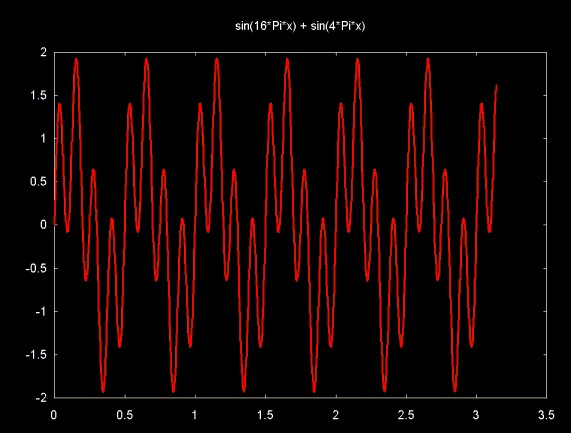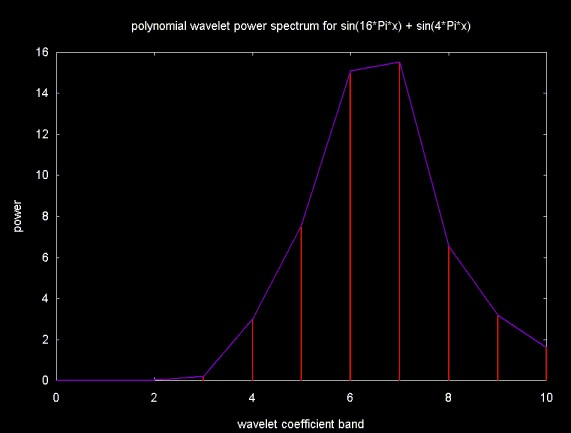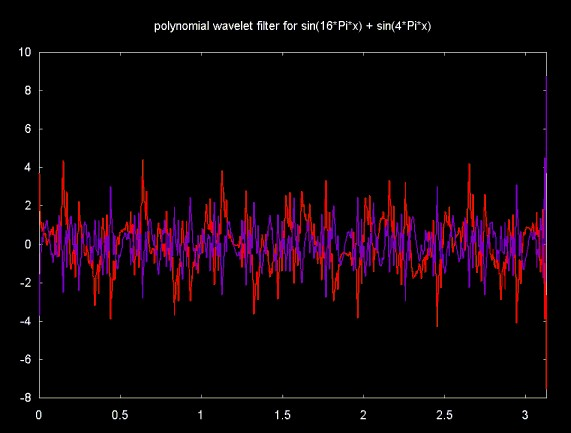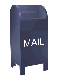## Spectral Analysis and Filtering with a Polynomial Interpolation Wavelet

The Haar wavelet algorithm operates on pairs of data points (even/odd) values. The Haar wavelet can miss detail (change) that occurs between the odd value of one pair and the even value of another pair. In contrast, an algorithm like Daubechies D4 catches this detail, since the wavelet is calculated on four points and shifted two points in each iteration.

I was looking for a wavelet algorithm that would catch detail that was missed by the Haar wavelet. In my search I read Wim Sweldens Building Your Own Wavelets at Home (see reference below), which describes a polynomial interpolation wavelet. This wavelet uses four point polynomial interpolation. As with any wavelet which is calculated on more than two points, special cases exist at the edges ot the time series (for a discussion of edge condition issues, see A Linear Algebra View of the Wavelet Transform). The polynomial interpolation wavelet interpolates points at the edges. However, polynomial interpolation is notorious for producing poorly behaved results for some combinations of points. This can certainly be seen when the wavelet is applied to the signal shown in Figure 1.

Figure 1The wavelet power spectrum plot for the polynomial interpolatoin wavelet is shown in Figure 2. This plot suggests that the polynomial interpolation wavelet does not clearly resolve the signal components. What is surprising is that band 9, which reflects the first order differences between the wavelet function and the actual data is relatively large (compared to the Daubechies D4 wavelet applied to the same data). This is surprising, since we might have expected polynomial interpolation to do well in a piece-wise fit of this signal.

Figure 2Figure 3 shows the two signals that result from building a low pass filter from wavelet bands 1 ... 6 and a high pass filter from bands 7 ... 9. The reconstruction of the component signals is poor, even worse than the Haar wavelet.

Figure 3Two implementations of the polynomial interpolation wavelet can be found in the source code that can be down loaded from the parent web page The wavelet in poly.h was used to generate these results. The polyHaar.h algorithm produced even worse results.

The wavelet power spectrum and the filter results shown here suggest that the polynomial interpolation wavelet may be a poor choice for most (or all) applications. Certainly for this signal, the wavelet performs very poorly. This result does show the usefulness of the wavelet power spectrum for analyzing the behavior of a wavelet function on a data set.

### References

• Ripples in Mathematics: The Discrete Wavelet Transform by A. Jensen and A. la Cour-Harbo, Springer, 2001. mine. here.

• Building Your Own Wavelets at Home by Wim Sweldens and Peter Schroder

Building Your Own Wavelets at Home is part of a course on Wavelets in Computer Graphics given at SigGraph 1994, 1995 and 1996.

Ian Kaplan
August 2002
Revised: